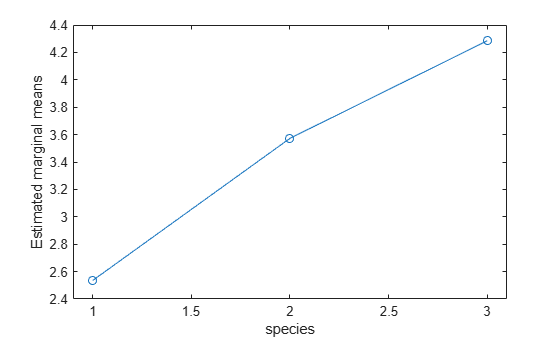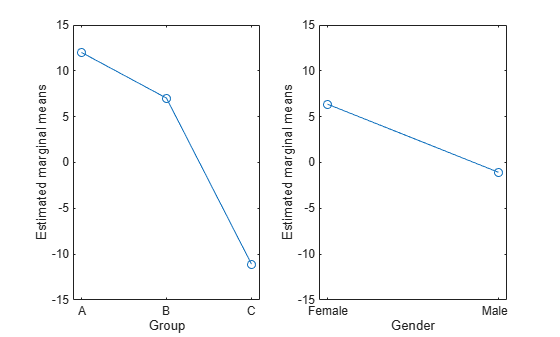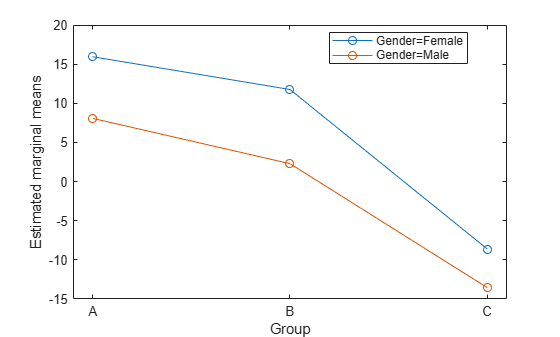plotprofile

Class: RepeatedMeasuresModel

Plot expected marginal means with optional grouping

Description

example

plotprofile(rm,X) plots the expected marginal means computed from the repeated measures model rm as a function of the variable X.

example

plotprofile(rm,Name,Value) plots the expected marginal means computed from the repeated measures model rm with additional options specified by one or more Name,Value pair arguments.

For example, you can specify the factors to group by or change the line colors.

H = plotprofile(___) returns handles, H, to the plotted lines.

Input Arguments

expand all

Repeated measures model, returned as a RepeatedMeasuresModel object.

For properties and methods of this object, see RepeatedMeasuresModel.

Name of a between-subjects or within-subjects factor, specified as a character vector or string scalar.

For example, if you want to plot the marginal means as a function of the groups of a between-subjects variable drug, you can specify it as follows.

Example: 'Drug'

Data Types: char | string

Name-Value Arguments

Specify optional comma-separated pairs of Name,Value arguments. Name is the argument name and Value is the corresponding value. Name must appear inside quotes. You can specify several name and value pair arguments in any order as Name1,Value1,...,NameN,ValueN.

Name of between-subject factor or factors, specified as the comma-separated pair consisting of 'Group' and a character vector, string array, or cell array of character vectors. This name-value pair argument groups the lines according to the factor values.

For example, if you have two between-subject factors, drug and sex, and you want to group the lines in the plot according to them, you can specify these factors as follows.

Example: 'Group',{'Drug','Sex'}

Data Types: char | string | cell

Marker to use for each group, specified as the comma-separated pair consisting of 'Marker' and a string array or cell array of character vectors.

For example, if you have two between-subject factors, drug and sex, with each having two groups, you can specify o as the marker for the groups of drug and x as the marker for the groups of sex as follows.

Example: 'Marker',{'o','o','x','x'}

Data Types: string | cell

Color for each group, specified as the comma-separated pair consisting of 'Color' and a character vector, string array, cell array of character vectors, or rows of a three-column RGB matrix.

For example, if you have two between-subject factors, drug and sex, with each having two groups, you can specify red as the color for the groups of drug and blue as the color for the groups of sex as follows.

Example: 'Color','rrbb'

Data Types: single | double | char | string | cell

Line style for each group, specified as the comma-separated pair consisting of 'LineStyle' and a string array or cell array of character vectors.

For example, if you have two between-subject factors, drug and sex, with each having two groups, you can specify - as the line style of one group and : as the line style for the other group as follows.

Example: 'LineStyle',{'-' ':' '-' ':'}

Data Types: string | cell

Output Arguments

expand all

Handle to plotted lines, returned as a handle.

Examples

expand all

The column vector species consists of iris flowers of three different species: setosa, versicolor, and virginica. The double matrix meas consists of four types of measurements on the flowers: the length and width of sepals and petals in centimeters, respectively.

Store the data in a table array.

t = table(species,meas(:,1),meas(:,2),meas(:,3),meas(:,4),...
'VariableNames',{'species','meas1','meas2','meas3','meas4'});
Meas = dataset([1 2 3 4]','VarNames',{'Measurements'});

Fit a repeated measures model, where the measurements are the responses and the species is the predictor variable.

rm = fitrm(t,'meas1-meas4~species','WithinDesign',Meas);

Perform data grouped by the factor species.

plotprofile(rm,'species')The estimated marginal means seem to differ with group. You can compute the standard error and the 95% confidence intervals for the marginal means using the margmean method.

The table between includes the between-subject variables age, IQ, group, gender, and eight repeated measures y1 through y8 as responses. The table within includes the within-subject variables w1 and w2. This is simulated data.

Fit a repeated measures model, where the repeated measures y1 through y8 are the responses, and age, IQ, group, gender, and the group-gender interaction are the predictor variables. Also specify the within-subject design matrix.

rm = fitrm(between,'y1-y8 ~ Group*Gender + Age + IQ','WithinDesign',within);

Plot the estimated marginal means based on the factors Group and Gender.

ax1 = subplot(1,2,1);
plotprofile(rm,'Group')
ax2 = subplot(1,2,2);
plotprofile(rm,'Gender')Plot the estimated marginal means based on the factor Group and grouped by Gender.

figure()
plotprofile(rm,'Group','Group','Gender')